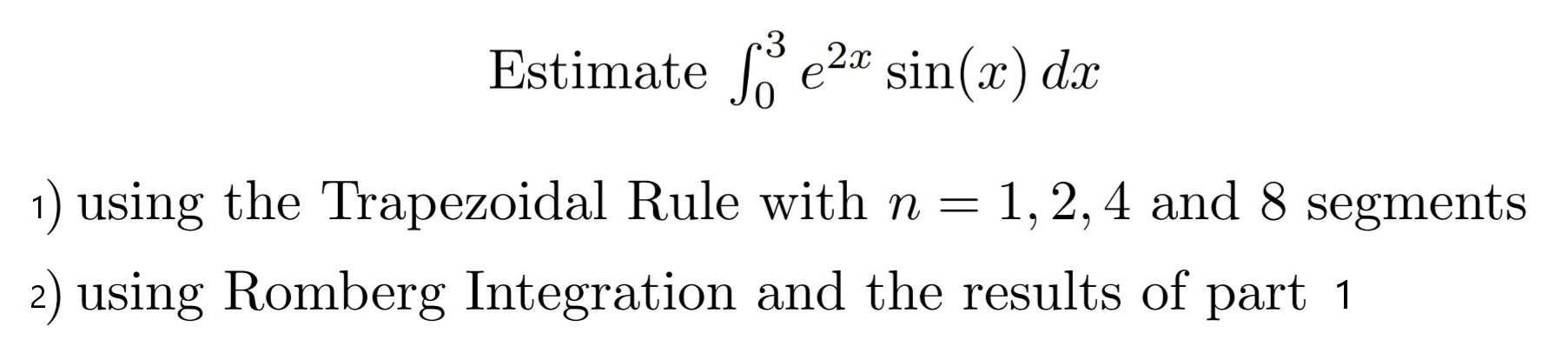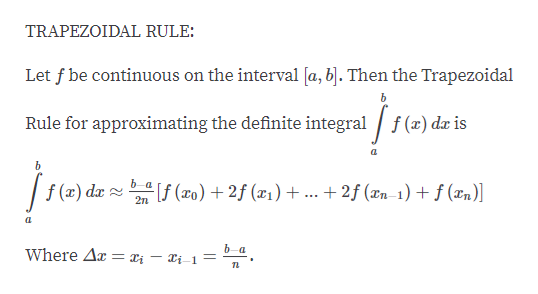# 3Estimatee2* sin(x) dx1 using the Trapezoidal Rule with n =1,2,4 and 8 segments2) using Romberg Integration and the results of part 1

Questionhelp_outlineImage Transcriptionclose3 Estimate e2* sin(x) dx 1 using the Trapezoidal Rule with n = 1,2,4 and 8 segments 2) using Romberg Integration and the results of part 1 fullscreen
check_circleExpert Solution
Step 1

Hey, since there are multiple subparts posted, we will answer the first questions. If you want any specific question to be answered, then please submit that question only or specify the question number in your message

Step 2

Given a function

Step 3

Note:

...help_outlineImage TranscriptioncloseTRAPEZOIDAL RULE: Let f be continuous on the interval [a, bj. Then the Trapezoidal Rule for approximating the definite integral f(x) dx is f(a) da f(o)2(x1)+ ... + 2f (Xn 1) + f (xn)] 2n a b-а Where Ar = Xi - Ii-1 = fullscreen

### Want to see the full answer?

See Solution

#### Want to see this answer and more?

Solutions are written by subject experts who are available 24/7. Questions are typically answered within 1 hour*

See Solution
*Response times may vary by subject and question
Tagged in

### Calculus